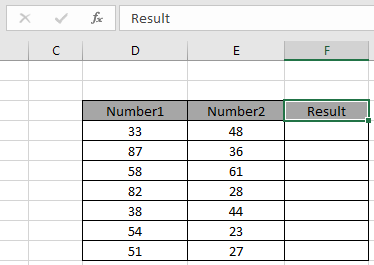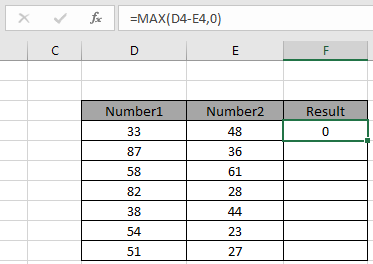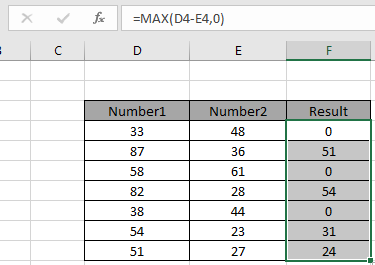# Replace negative numbers with 0 value in Excel

In this article, we will learn about how to count valid numbers in a list in Excel.

Scenario:
At Instance, we need to calculate some values with formula and need no negative numbers in response. Replace negative numbers with 0 value using formula in excel explained here with an example.

How to solve the problem.
We will construct a formula using the MAX function in Excel. Here we need to first need to know what operations are performing and how to change the negative result to 0 value. Use the MAX function to get what we want.
Use the formula:

= MAX ( operation, 0)

Variables:

operation: perform a formula inside the MAX function as argument.

0 : value given as a direct argument.

Example:

All of these might be confusing to understand. So, let's test this formula via running it on the example shown below.

Here for example we have two ranges of numbers as Number1 & Number2. We need to operate subtraction Number1 from Number2 and need no negative numbers in response. Replace the negative number with 0 value.We need to use the formula for the above stated problem.

Use the formula:

= MAX ( D4-E4 , 0 )

Explanation:

1. D4-E4 gets the subtraction result value in D4 cell from value in E4 cell.
2. The subtraction 33 - 48 returns -15 value.
3. MAX( -15, 0 ), MAX function returns the maximum of the two numbers which is 0.
4. The function returns the number if it is greater than 0.As you can see the formula returns 0 as result because the operation returns a negative number. Now copy and paste the formula from 1st result value to the rest of the cells.As you can see you can result in the Result column, we get obtained the only positive numbers excluding negative values. Negative values are replaced by value 0 using the above explained formula.

Here are some observational notes as mentioned below.

Notes:

1. Use the cell reference method for the input argument. 0 value can also be given as cell reference
2. Operation performed inside the function can be customized.
3. The inside operation must result in a number value or else the formula returns error.

Hope you understood how to Replace negative numbers with 0 value in Excel. Explore more articles on Excel formulas on numbers here. Please feel free to state your query or feedback for the above article. We will assist you.

Related Articles

COUNTIFS with Dynamic Criteria Range : Count cells dependent on other cell values in Excel.

COUNTIFS Two Criteria Match : Count cells matching two different criteria on list in excel.

COUNTIFS With OR For Multiple Criteria : Count cells having multiple criteria match using the OR function in Excel.

The COUNTIFS Function in Excel : Count cells dependent on other cell values in Excel.

How to Use Countif in VBA in Microsoft Excel : Count cells using Visual Basic for Applications code in Excel.

How to use wildcards in excel : Count cells matching phrases using the wildcards in excel.

Popular Articles

50 Excel Shortcut to Increase Your Productivity

Edit a dropdown list

If with conditional formatting

If with wildcards

Vlookup by date

Convert Inches To Feet and Inches in Excel 2016

Join first and last name in excel

Count cells which match either A or B

Terms and Conditions of use

The applications/code on this site are distributed as is and without warranties or liability. In no event shall the owner of the copyrights, or the authors of the applications/code be liable for any loss of profit, any problems or any damage resulting from the use or evaluation of the applications/code.